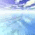## Friday, June 6, 2014

### stone flakes

I browsed through UC Irvine Machine Learning Repository! the other day and noticed a nice data set regarding stone flakes produced by our ancestors, the prehistoric men. To quote the dataset owners:
'The data set concerns the earliest history of mankind. Prehistoric men created the desired shape of a stone tool by striking on a raw stone, thus splitting off flakes, the waste products of the crafting process. Archaeologists do not find many tools, but they do find flakes. The data set is about these flakes.' The question attached to the data is: 'Does the data reflect the technological progress during several hundred thousand years?'. This blog post does not tackle that question but first examines the data as multivariate set.

### Data

The data consists of two data sets; one set for flake properties, where rows do not stand for single flakes but for whole inventories of them. The annotation data are associated properties, such as age and hominid type. There are 79 records. As can be seen for the first records, there are some missing data.
ID  LBI  RTI  WDI FLA  PSF  FSF ZDF1 PROZD
1  ar   NA 35.3 2.60  NA 42.4 24.2 47.1    69
2 arn 1.23 27.0 3.59 122  0.0 40.0 40.0    30
3  be 1.24 26.5 2.90 121 16.0 20.7 29.7    72
4 bi1 1.07 29.1 3.10 114 44.0  2.6 26.3    68
5 bi2 1.08 43.7 2.40 105 32.6  5.8 10.7    42
6 bie 1.39 29.5 2.78 126 14.0  0.0 50.0    78
ID group  age dating mat region site number
1  ar     3 -120    geo   2      d    0     34
2 arn     2 -200   typo   1    mit    1      5
3  be     2 -200   typo   1    mit    1    331
4 bi1     1 -300    geo   1    mit    0   4111
5 bi2     1 -300    geo   2    mit    0     77
6 bie     2 -200    geo   1    mit    1      8

### Density plots

To get an idea about the data I have made density plots. For compactness lattice plots are used. The reshape is just a preparation for that. From data perspective, the homo sapiens group is pretty small has a small data range.

r12 <- merge(r1,r2)
# density
cols <- colorRampPalette(c('violet','gold','seagreen'))(4)
library(lattice)
long <- reshape(r12,direction='long',
v.names='Response',
varying=list(colnames(r2)[-1]),
idvar=c('ID','group'),
timevar='Variable',
times=colnames(r2)[-1])
densityplot(~ Response | Variable ,groups= group,
data=long ,scales=list(relation='free'),
col=cols,
auto.key=list(
text=c('Lower Paleolithic, Homo ergaster?, oldest',
'Levallois technique',
'Middle Paleolithic, probably Neanderthals',
'Homo Sapiens, youngest'),
col=cols,
lines=FALSE))

### Biplot

A bipot is easily made. However, I am a bit of a fan of the biplots detailed in Gower and Hand's book. Since the heavy lifting for that is now in package calibrate they are easily made.
r12c <- r12[complete.cases(r12),]
pr1 <- princomp(~ LBI + RTI + WDI + FLA + PSF + FSF + ZDF1 + PROZD,
r12c,
cor=TRUE,
scores=TRUE)
biplot(pr1,xlabs=r12c\$ID)
Most of the following code is from calibrate's vignette. The colors in point labels are an annotation which I made. Unfortunately the textxy() function did not get color as I intended, so a for loop is made to get it correct. The length of the blue axis are made via trial and error. It should be noted that, similar to any biplot, there is some deformation, the axis are approximate.
library(calibrate)
X0<- subset(r12c,,c(LBI,RTI,WDI,FLA,PSF,FSF,ZDF1 ,PROZD))
X <- scale(X0)
rownames(X) <- r12c\$ID
pca.results <- princomp(X,cor=FALSE)
Fp <- pca.results\$scores
# no margins
par(mar=rep(0.05,4))
plot(Fp[,1],Fp[,2],
pch=16,asp=1,
xlim=c(-5,5),ylim=c(-5,5),
frame.plot=TRUE,axes=FALSE,
cex=0.5,type='n',
col=cols[r12c\$group])

for( ii in unique(r12c\$group))
textxy(Fp[r12c\$group==ii,1],
Fp[r12c\$group==ii,2],
rownames(X)[r12c\$group==ii],
cex=0.75,
col=cols[ii],offset=0)

for (ii in 1:ncol(X)) {
myseq <- seq(-2,2)
if (colnames(X)[ii]=='LBI') myseq <-seq(-2,3)
if (colnames(X)[ii] %in% c('RTI','FSF','PROZD')) myseq <-seq(-1.4,1.4)
if (colnames(X)[ii]=='ZDF1') myseq <-seq(-1.5,2)
ticklab <- pretty(myseq*attr(X,'scaled:scale')[ii]+attr(X,'scaled:center')[ii])

ticklabc <- (ticklab-attr(X,'scaled:center')[ii])/attr(X,'scaled:scale')[ii]
yc <- X[,ii]
g <- Gs[ii,1:2]
Calibrate.X3 <- calibrate(g,yc,ticklabc,Fp[,1:2],ticklab,tl=0.1,
axislab=colnames(X)[ii],cex.axislab=0.75,where=1,labpos=4)
}

legend(x='topleft',
legend=c('Lower Paleolithic, Homo ergaster?, oldest',
'Levallois technique',
'Middle Paleolithic, probably Neanderthals',
'Homo Sapiens, youngest'),
text.col=cols,
ncol=1,cex=.75)

### Hierarchical clustering

In the clustering it was chosen to use scaled data, just like the biplot. The reason is that the scales of the variables is quite different. The distance used is simple Euclidian, with average linkage. The code for colors in the dendrogam is not standard, but extracted from stackoverflow.
ddi <- dist(X)
par(cex=.7)
hc <- hclust(ddi,method='average')

labelCol <- function(x) {
if (is.leaf(x)) {
## fetch label
label <- attr(x, "label")
## set label color to red for A and B, to blue otherwise
attr(x, "nodePar") <- list(lab.col=cols[r12\$group[r12\$ID==label]],
pch=46)
}
return(x)
}
## apply labelCol on all nodes of the dendrogram
dd <- dendrapply(as.dendrogram(hc,hang=.1), labelCol)

par(mar=c(3,.1,.1,2))
plot(dd,horiz=TRUE)

legend(x='topleft',
legend=c('Lower Paleolithic, Homo ergaster?, oldest',
'Levallois technique',
'Middle Paleolithic, probably Neanderthals',
'Homo Sapiens, youngest'),
text.col=cols,
ncol=1,cex=.75)

### Interpretation

It would seem the data shows that the flakes shapes give a reasonable display of the groups, without using these groups as input information. This suggests that there is indeed a relation between flakes shape and time, which is for a future blog post.

1.2.